# TS 4273 Traffic Engineering Traffic Stream Characteristics Traffic

• Slides: 87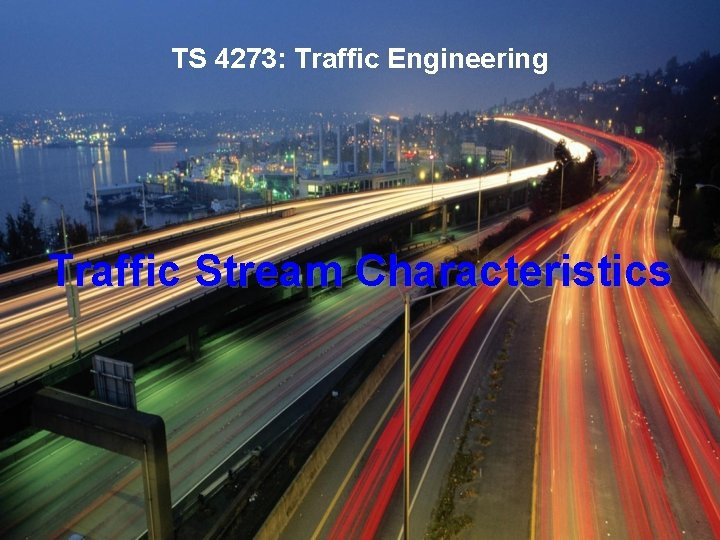TS 4273: Traffic Engineering Traffic Stream Characteristics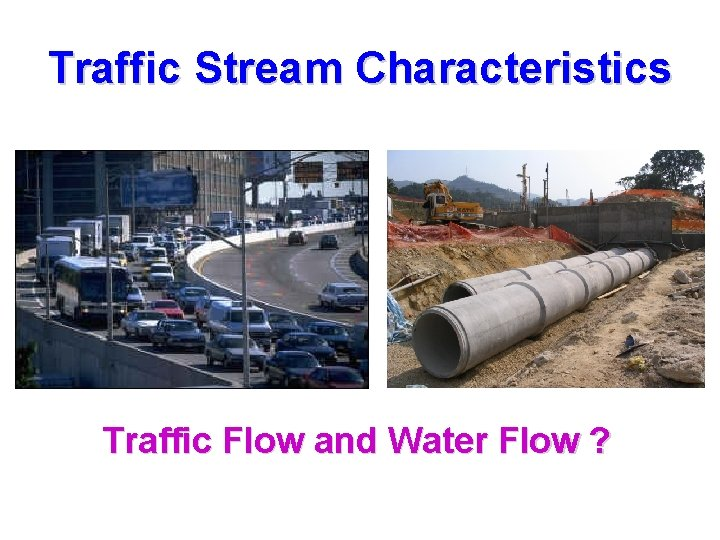Traffic Stream Characteristics Traffic Flow and Water Flow ?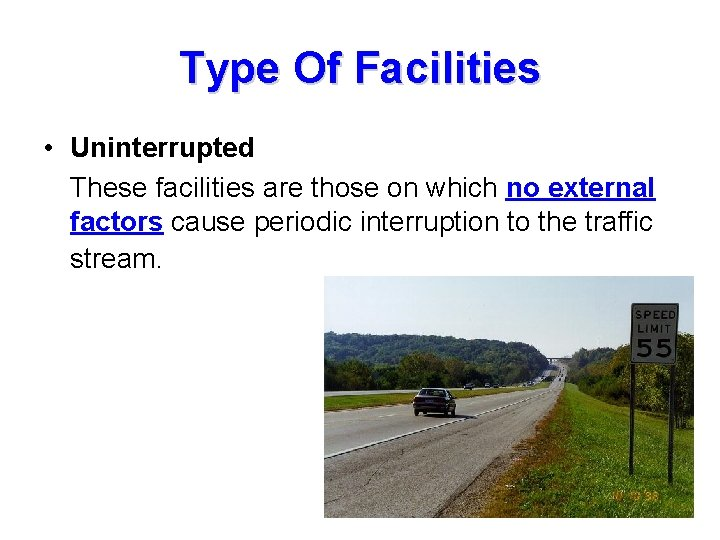Type Of Facilities • Uninterrupted These facilities are those on which no external factors cause periodic interruption to the traffic stream.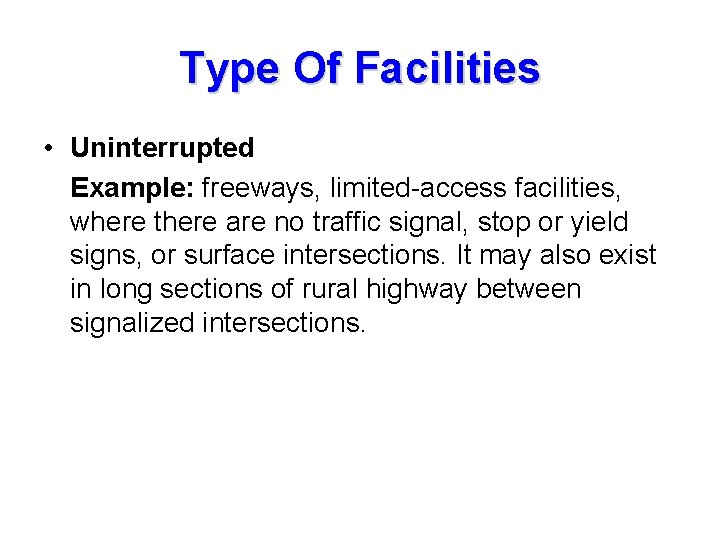Type Of Facilities • Uninterrupted Example: freeways, limited-access facilities, where there are no traffic signal, stop or yield signs, or surface intersections. It may also exist in long sections of rural highway between signalized intersections.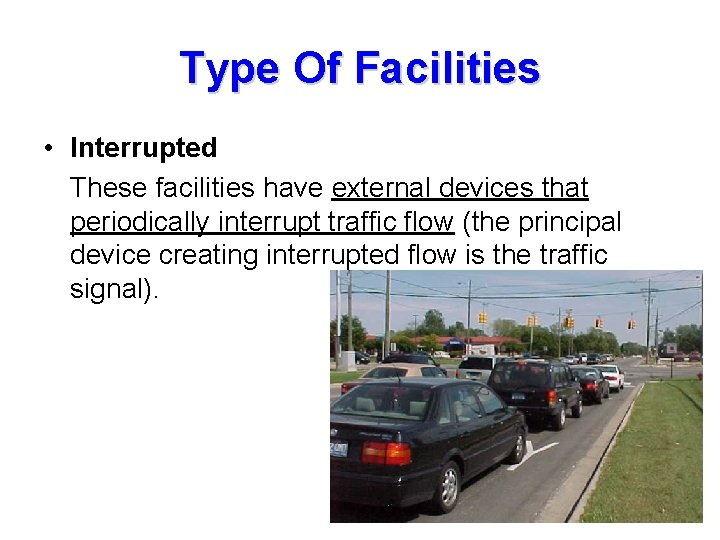Type Of Facilities • Interrupted These facilities have external devices that periodically interrupt traffic flow (the principal device creating interrupted flow is the traffic signal).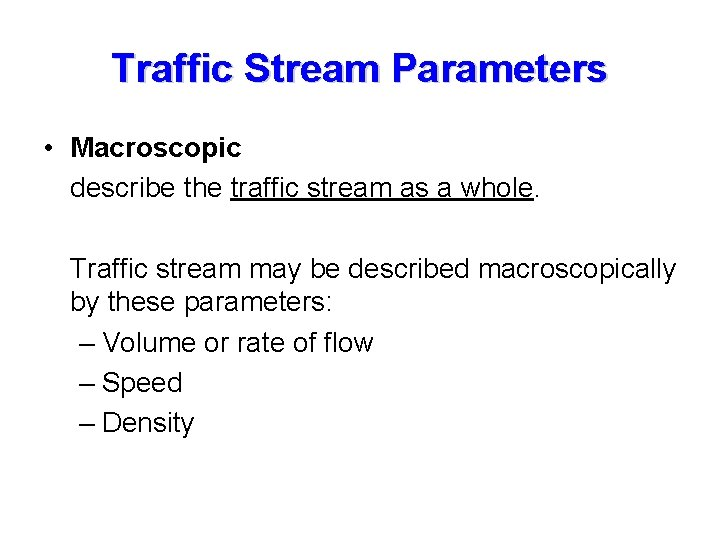Traffic Stream Parameters • Macroscopic describe the traffic stream as a whole. Traffic stream may be described macroscopically by these parameters: – Volume or rate of flow – Speed – Density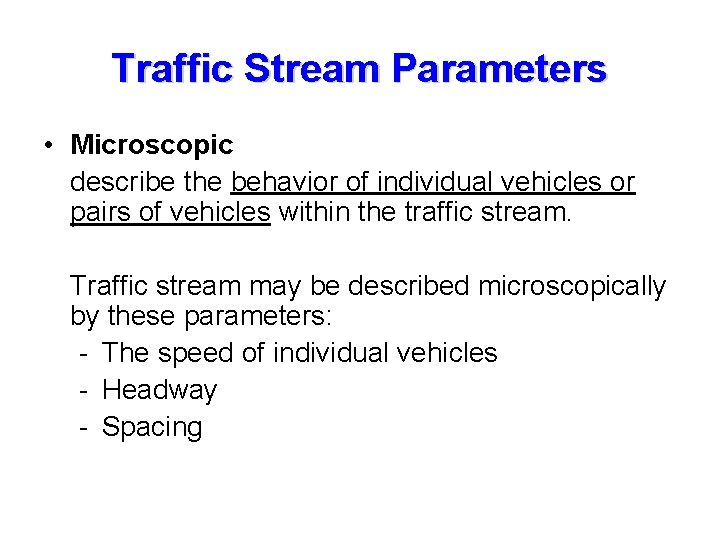Traffic Stream Parameters • Microscopic describe the behavior of individual vehicles or pairs of vehicles within the traffic stream. Traffic stream may be described microscopically by these parameters: - The speed of individual vehicles - Headway - SpacingVolume Patterns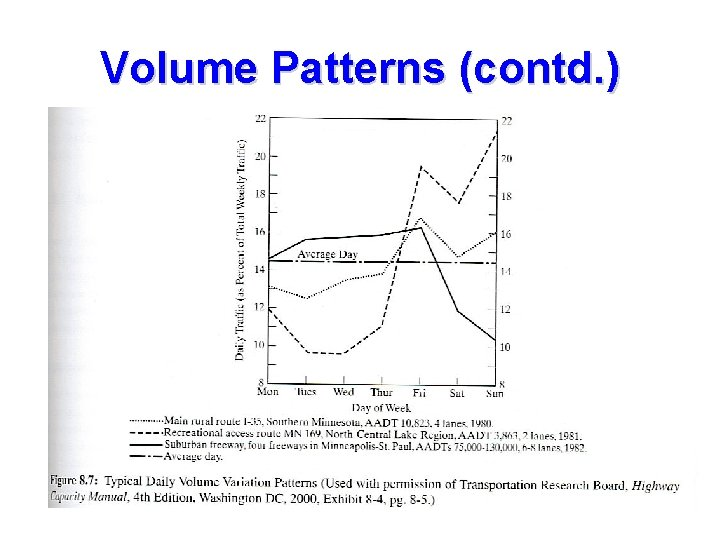Volume Patterns (contd. )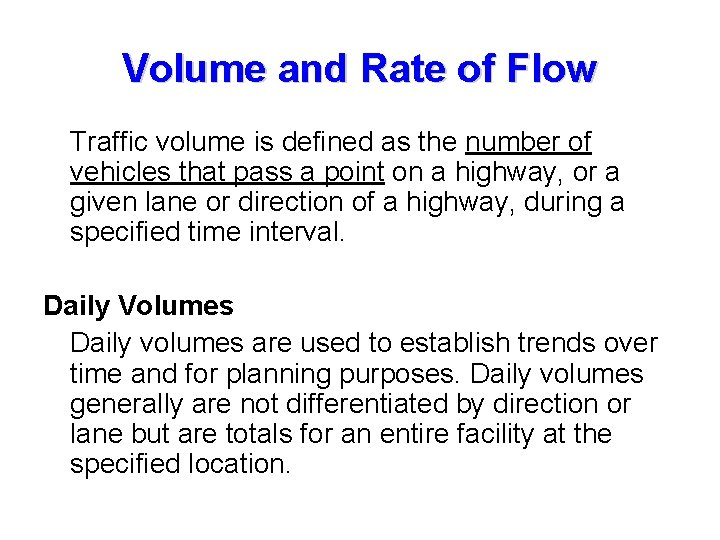Volume and Rate of Flow Traffic volume is defined as the number of vehicles that pass a point on a highway, or a given lane or direction of a highway, during a specified time interval. Daily Volumes Daily volumes are used to establish trends over time and for planning purposes. Daily volumes generally are not differentiated by direction or lane but are totals for an entire facility at the specified location.Volume and Rate of Flow There are four daily volume parameters that are widely used in traffic engineering: - Annual Average Daily Traffic (AADT) - Annual Average Weekday Traffic (AAWT) - Average Daily Traffic (ADT) - Average Weekday Traffic (AWT) All of these volumes are stated in terms of vehicles per day (vpd).Daily Volumes - Annual Average Daily Traffic (AADT): is the average 24 hour traffic volume at a given location over a full 365 -day year – that is the total number of vehicles passing the site in a year divided by 365. - Annual Average Weekday Traffic (AAWT): is the average 24 -hour traffic volume occurring on weekdays over a full year. AAWT is computed by dividing the total weekday traffic volume for the year by 260. This volume is of considerable interest where weekend traffic is light, so that averaging higher weekday volumes over 365 days would mask the impact of weekday traffic.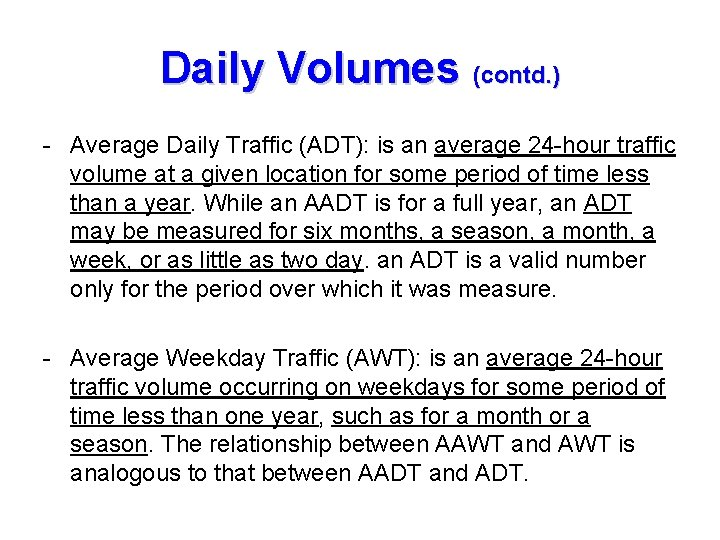Daily Volumes (contd. ) - Average Daily Traffic (ADT): is an average 24 -hour traffic volume at a given location for some period of time less than a year. While an AADT is for a full year, an ADT may be measured for six months, a season, a month, a week, or as little as two day. an ADT is a valid number only for the period over which it was measure. - Average Weekday Traffic (AWT): is an average 24 -hour traffic volume occurring on weekdays for some period of time less than one year, such as for a month or a season. The relationship between AAWT and AWT is analogous to that between AADT and ADT.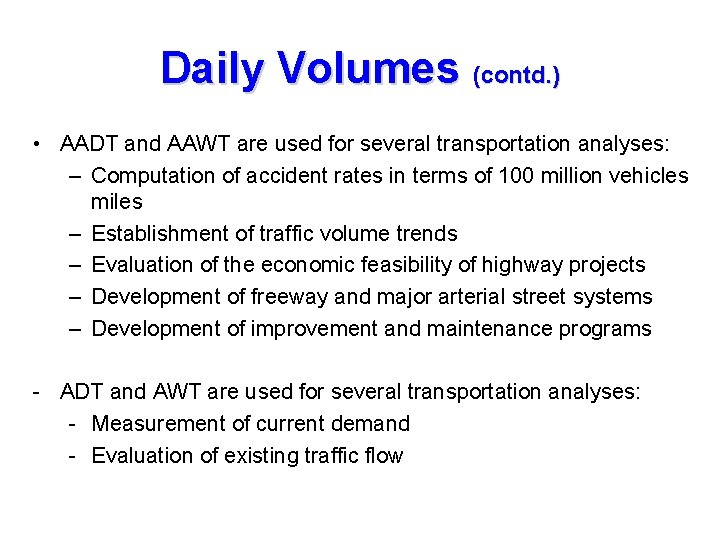Daily Volumes (contd. ) • AADT and AAWT are used for several transportation analyses: – Computation of accident rates in terms of 100 million vehicles miles – Establishment of traffic volume trends – Evaluation of the economic feasibility of highway projects – Development of freeway and major arterial street systems – Development of improvement and maintenance programs - ADT and AWT are used for several transportation analyses: - Measurement of current demand - Evaluation of existing traffic flow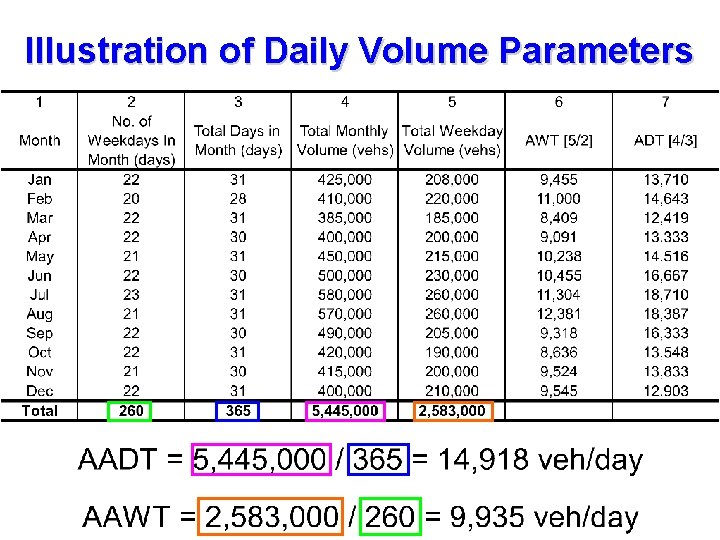Illustration of Daily Volume ParametersHourly Volumes Daily volumes, while useful for planning purposes, cannot be used alone for design or operational analysis purposes. Volume varies considerably over the 24 hours of the day, with periods of maximum flow occurring during the morning and evening commuter “rush hours”.Hourly Volumes The single hour of the day that has the highest hourly volume is referred to as the “peak hour”. The traffic volume within this hour is of greatest interest to traffic engineers for design and operational analysis usage. The peak-hour volume is generally stated as a directional volume (each direction of flow is counted separately).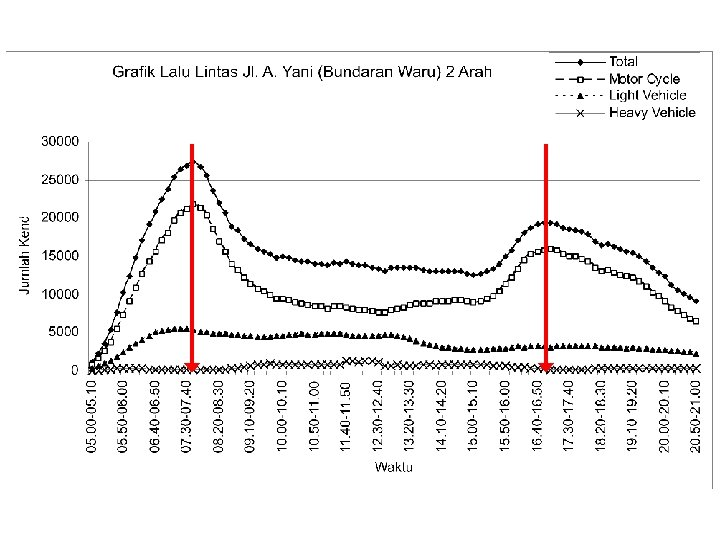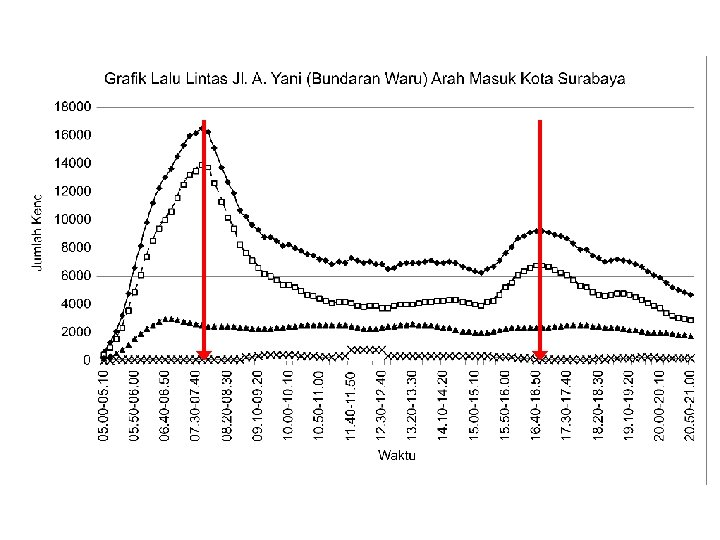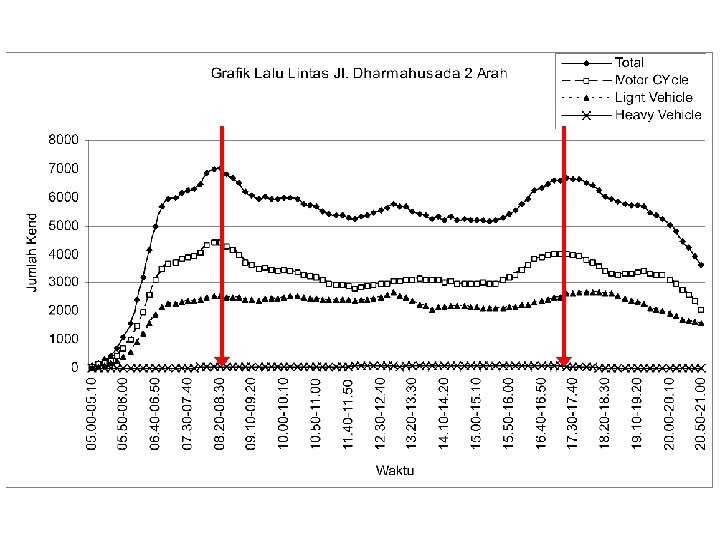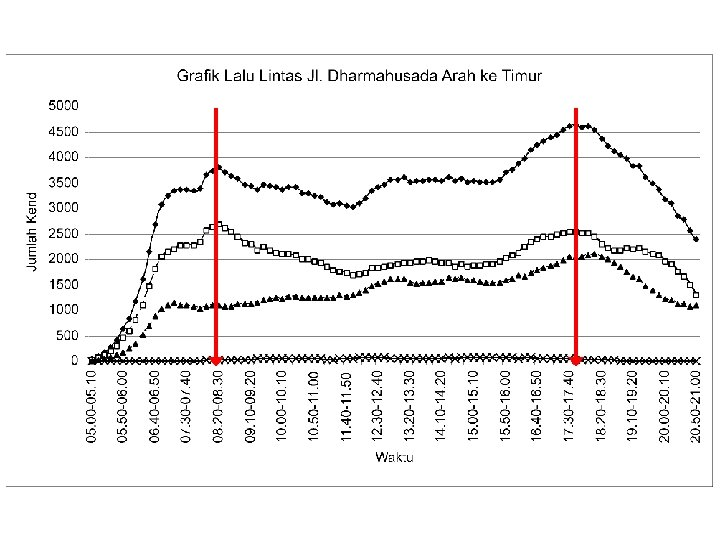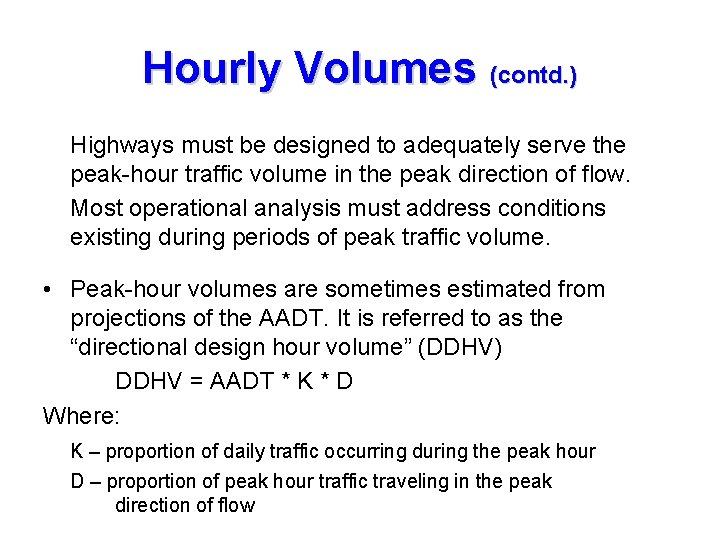Hourly Volumes (contd. ) Highways must be designed to adequately serve the peak-hour traffic volume in the peak direction of flow. Most operational analysis must address conditions existing during periods of peak traffic volume. • Peak-hour volumes are sometimes estimated from projections of the AADT. It is referred to as the “directional design hour volume” (DDHV) DDHV = AADT * K * D Where: K – proportion of daily traffic occurring during the peak hour D – proportion of peak hour traffic traveling in the peak direction of flowHourly Volumes (contd. ) • Used for several transportation analyses: – Functional classification of roads – Design of geometric characteristics of highways (number of lanes) – Capacity analysis – Development of programs related to traffic operations – Development of parking regulations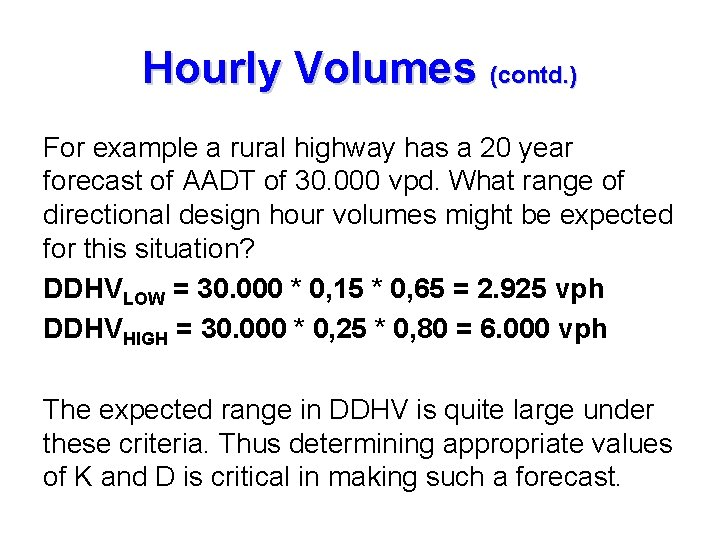Hourly Volumes (contd. ) For example a rural highway has a 20 year forecast of AADT of 30. 000 vpd. What range of directional design hour volumes might be expected for this situation? DDHVLOW = 30. 000 * 0, 15 * 0, 65 = 2. 925 vph DDHVHIGH = 30. 000 * 0, 25 * 0, 80 = 6. 000 vph The expected range in DDHV is quite large under these criteria. Thus determining appropriate values of K and D is critical in making such a forecast.Sub Hourly Volumes While hourly traffic volumes form the basis for many forms of traffic design and analysis, the variation of traffic within given hour is also of considerable interest. The quality of traffic flow is often related to shortterm fluctuations in traffic demand. A facility may have sufficient capacity to serve the peak-hour demand, but short-term peaks of flow within the hour may exceed capacity and create breakdown.Sub Hourly Volumes (cont’d)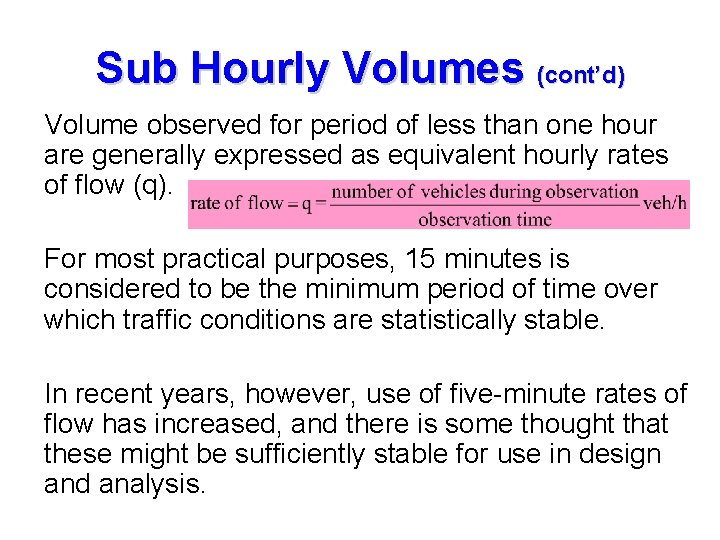Sub Hourly Volumes (cont’d) Volume observed for period of less than one hour are generally expressed as equivalent hourly rates of flow (q). For most practical purposes, 15 minutes is considered to be the minimum period of time over which traffic conditions are statistically stable. In recent years, however, use of five-minute rates of flow has increased, and there is some thought that these might be sufficiently stable for use in design and analysis.Sub Hourly Volumes (cont’d) PHF (Peak Hour Factor): defines the relationship between the hourly volume and the maximum rate of flow within the hour. PHF = Hourly volume / maximum rate of flow For standard 15 -minute analysis period, this become: PHF = Hourly volume / (4 * maximum 15 -minute volume within the hour)Sub hourly Volumes (contd. ) Peak-Hour Factor (PHF): is the ratio of the volume occurring during the peak hour to a maximum rate of flow during a given time period within the peak hour For standard 15 -minute analysis period, this become: Where, HV – Hourly Volume V 15 – Maximum 15 minute volume within the hourSub Hourly Volumes (contd. ) Example of volumes and rate of flow Time interval 5: 00 -5: 15 PM 5: 15 -5: 30 PM 5: 30 -5: 45 PM 5: 45 -6: 00 PM For the hour 5: 00 -6: 00 PM Volume Rate of flow (vehicles) (vehicles/h) 950 * 4 = 3. 800 1. 150 * 4 = 4. 600 1. 250 * 4 = 5. 000 1. 000 * 4 = 4. 000 4. 350 (veh/h) A facility may have capacity adequate to serve the peak-hour demand, but short-term peaks of flow within the peak hour may exceed capacity, thereby creating a breakdown.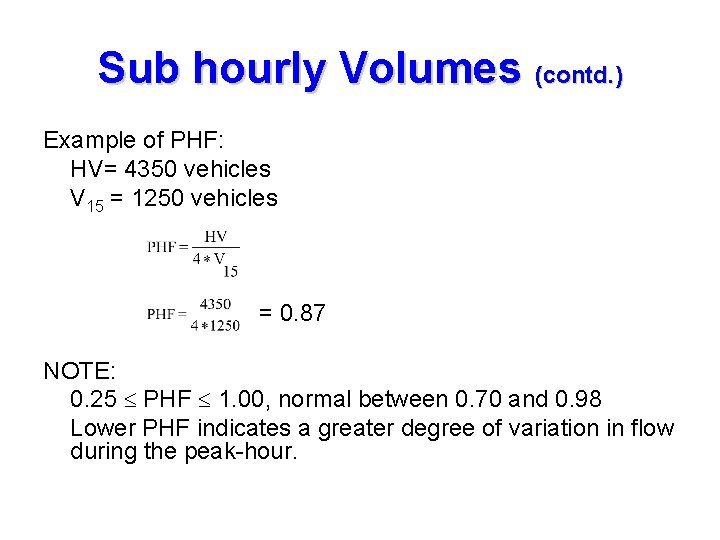Sub hourly Volumes (contd. ) Example of PHF: HV= 4350 vehicles V 15 = 1250 vehicles = 0. 87 NOTE: 0. 25 PHF 1. 00, normal between 0. 70 and 0. 98 Lower PHF indicates a greater degree of variation in flow during the peak-hour.Macroscopic Parameters • Flow (Q) – number of vehicles traversing a point of roadway per unit time (vehicles/hour) • Density (K) – number of vehicles occupying a given length of lane or roadway averaged over time (vehicles/mile) • Speed (U) – distance traversed by a vehicle per unit time (miles/hour)Q-K-U Relationship Flow, Q (veh/hr) = Density, K (veh/mile) x Speed, U (miles/hr) For example, say, Flow, Q = 1200 veh/hour Speed, U = 30 miles/hour Density, K = Q/U = 1200/30 = 40 veh/mileFundamental Diagram of Traffic Flow • • Density zero, flow also zero Density increases, flow also increases When density reached maximum (jam density), flow is equal to zero (car line up end to end) Density increases from zero, flows also increases up to a maximum value. After this value, density keeps increasing but flow decreases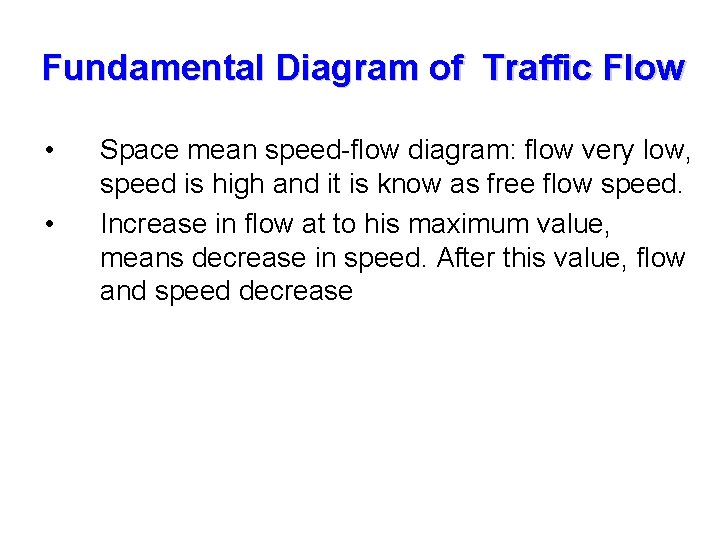Fundamental Diagram of Traffic Flow • • Space mean speed-flow diagram: flow very low, speed is high and it is know as free flow speed. Increase in flow at to his maximum value, means decrease in speed. After this value, flow and speed decreaseSpeed (miles/hr) Flow (veh/hr) Q-K-U Relationship Jam Density Critical Density Maximum Flow Critical Speed Critical Density (veh/mile) Flow (veh/hr)Traffic Stream Characteristics o Kepadatan 0, Volume 0, kecepatan bebas o Volume meningkat, hampir tidak ada hambatan o Volume maksimum, kepadatan meningkat, kecepatan menurun o Volume 0, kepadatan maksimum.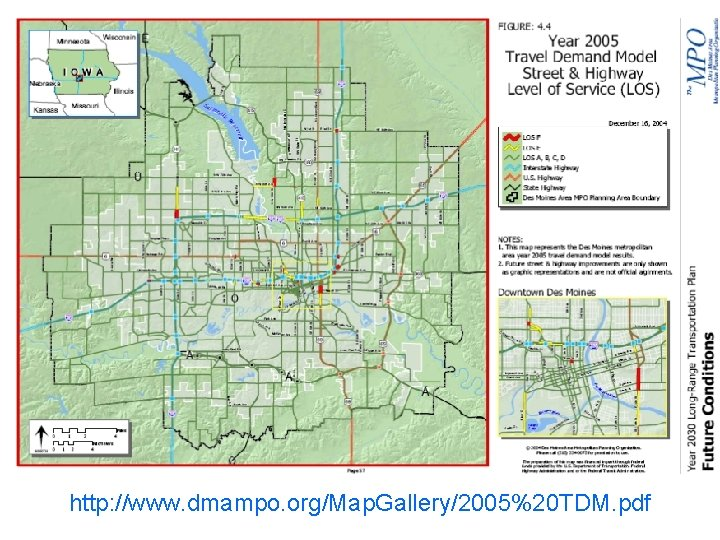http: //www. dmampo. org/Map. Gallery/2005%20 TDM. pdf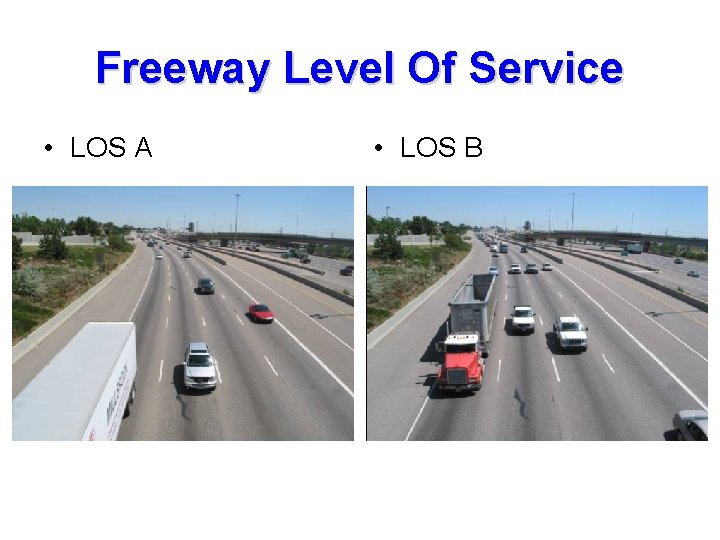Freeway Level Of Service • LOS A • LOS B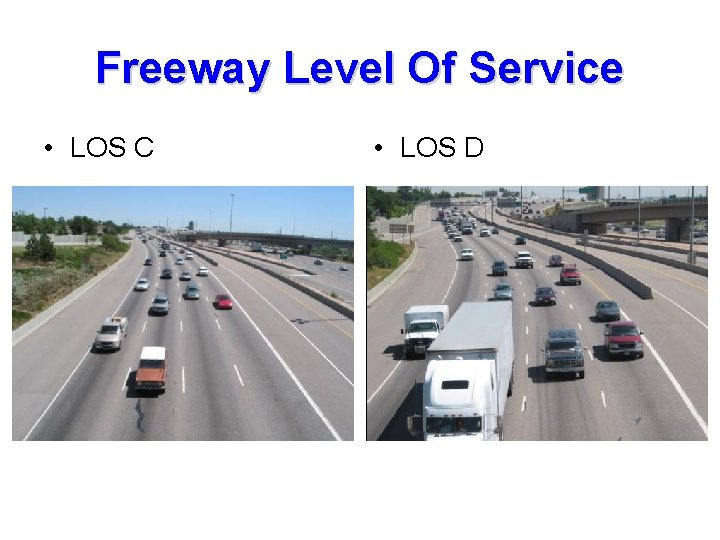Freeway Level Of Service • LOS C • LOS D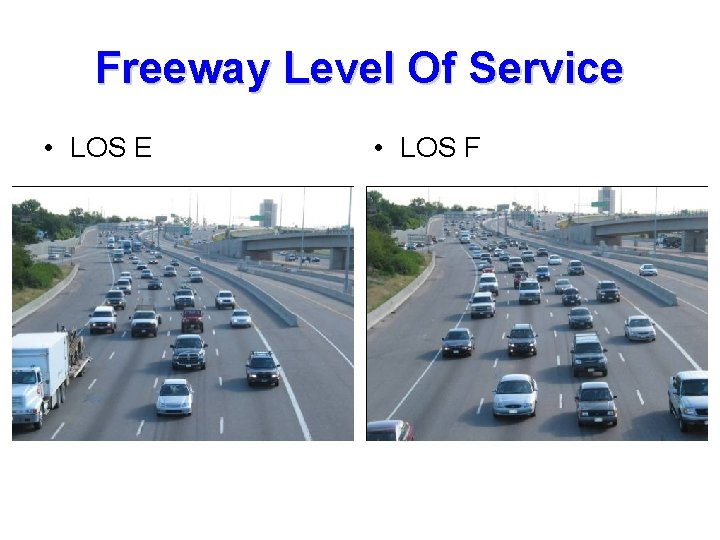Freeway Level Of Service • LOS E • LOS FLevel Of Service Untuk SelasarTraffic Stream Characteristics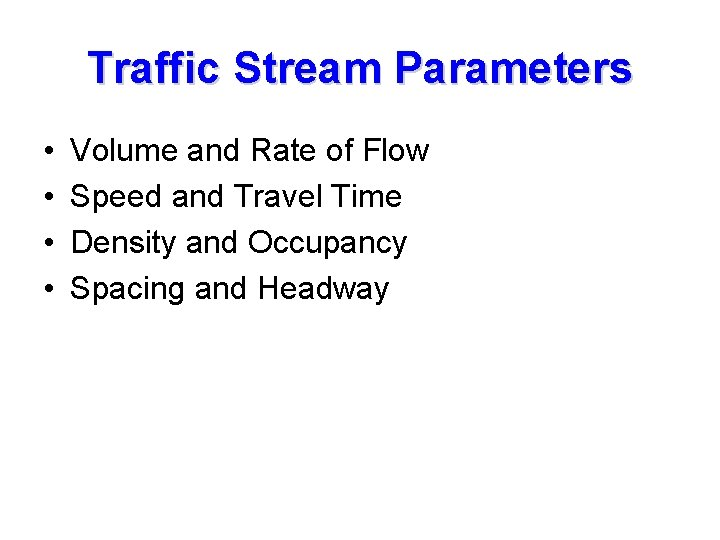Traffic Stream Parameters • • Volume and Rate of Flow Speed and Travel Time Density and Occupancy Spacing and HeadwaySPEED • Space Mean Speed • vs = average travel speed or space mean speed (kph) • L = length of the highway segment (km) • ti = travel time of the ith vehicle to cross the section (hours) • n = number of travel times observedSPEED • Space Mean Speed – Segment Length 1 km – Travel Time: • Vehicle A 45 seconds 0, 0125 hr/km 80 kph • Vehicle B 60 seconds 0, 0166 hr/km 60 kph • Vehicle C 72 seconds 0, 0200 hr/km 50 kph – What is the average travel speed of these three vehicles?SPEED • Space Mean Speed – Average Travel Time • [0, 0125 + 0, 0166 + 0, 0200] / 3 = 0, 016389 hr/km – Average Travel Speed • 1 / 0, 016389 = 61, 01695 61 kphSPEED • Time Mean Speed • vs = time mean speed (kph) • vi = spot speed (kph) • n = number of travel times observed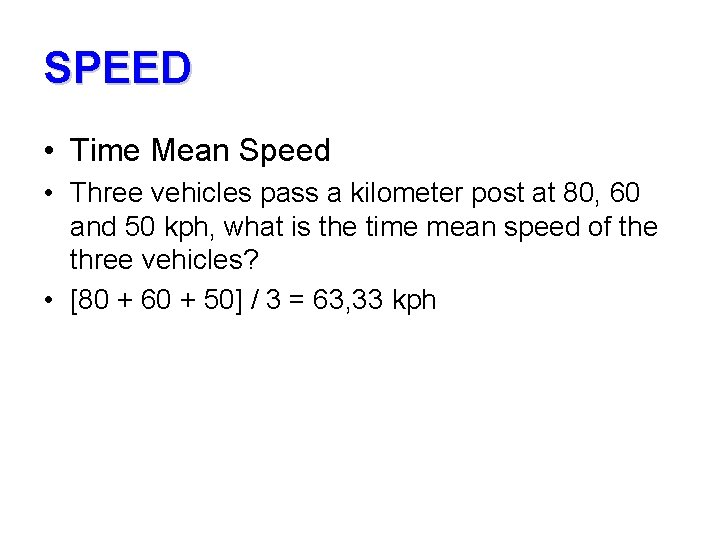SPEED • Time Mean Speed • Three vehicles pass a kilometer post at 80, 60 and 50 kph, what is the time mean speed of the three vehicles? • [80 + 60 + 50] / 3 = 63, 33 kphSPEED • Time Mean Speed (TMS) Kecepatan individu yang melintas suatu segmen selama periode waktu tertentu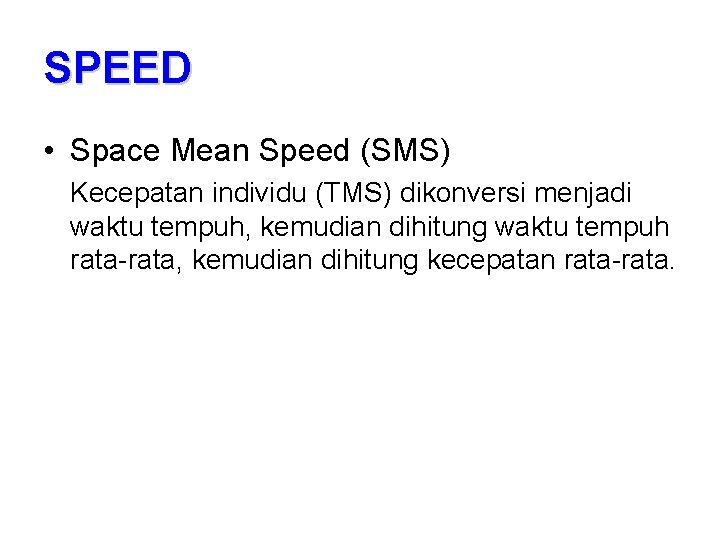SPEED • Space Mean Speed (SMS) Kecepatan individu (TMS) dikonversi menjadi waktu tempuh, kemudian dihitung waktu tempuh rata-rata, kemudian dihitung kecepatan rata-rata.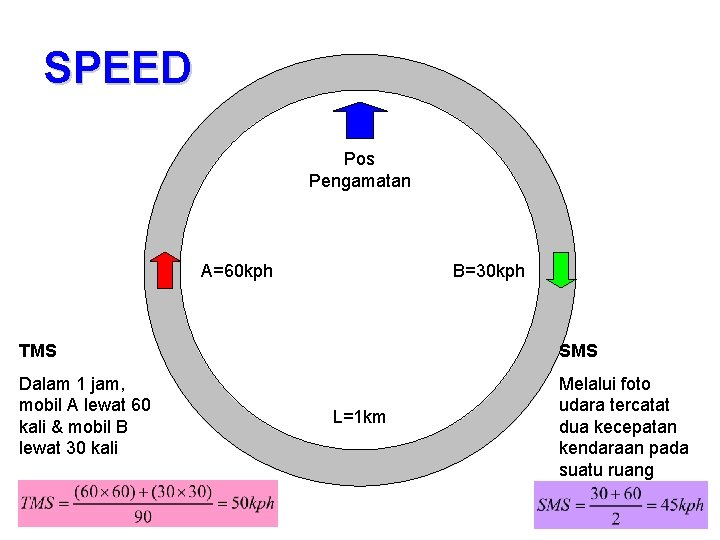SPEED Pos Pengamatan B=30 kph A=60 kph TMS SMS Dalam 1 jam, mobil A lewat 60 kali & mobil B lewat 30 kali Melalui foto udara tercatat dua kecepatan kendaraan pada suatu ruang L=1 km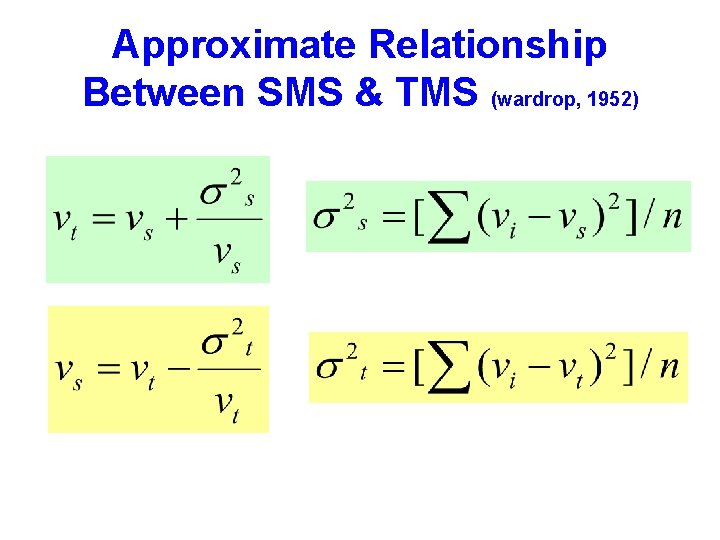Approximate Relationship Between SMS & TMS (wardrop, 1952)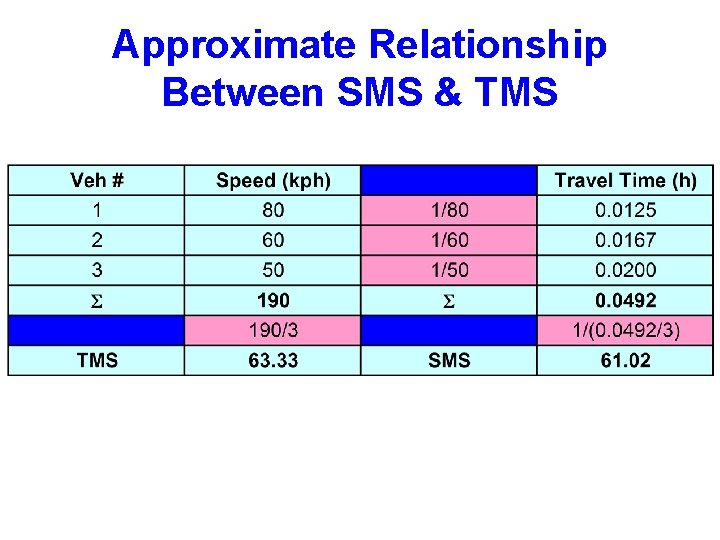Approximate Relationship Between SMS & TMSApproximate Relationship Between SMS & TMSApproximate Relationship Between SMS & TMS (wardrop, 1952)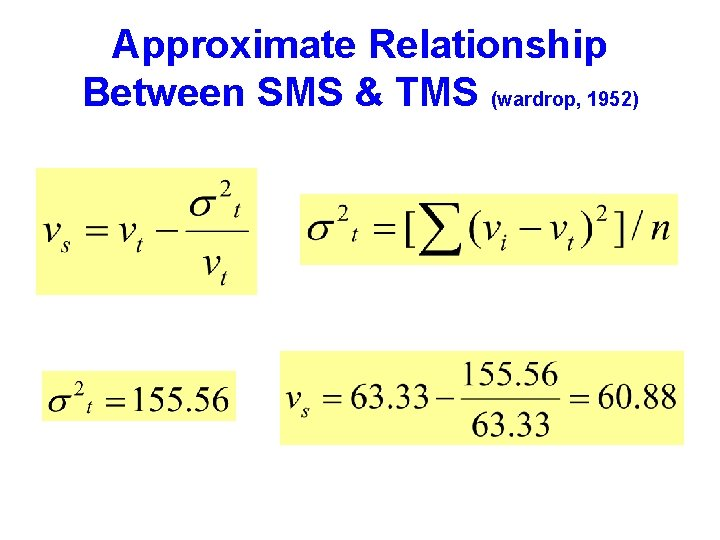Approximate Relationship Between SMS & TMS (wardrop, 1952)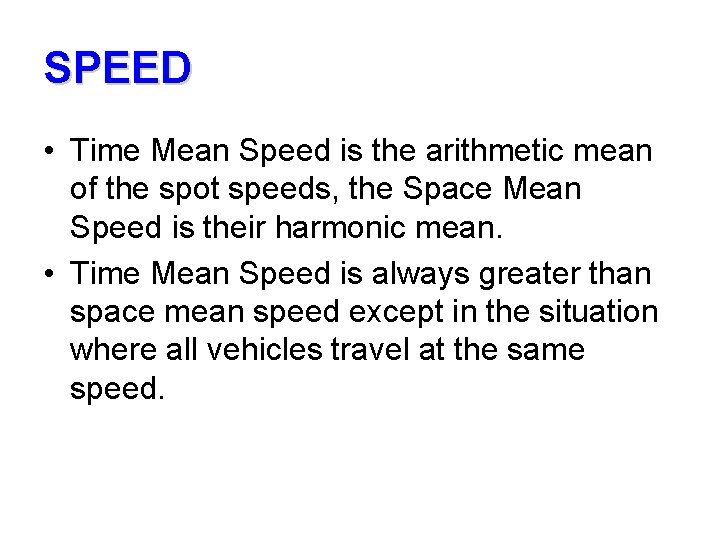SPEED • Time Mean Speed is the arithmetic mean of the spot speeds, the Space Mean Speed is their harmonic mean. • Time Mean Speed is always greater than space mean speed except in the situation where all vehicles travel at the same speed.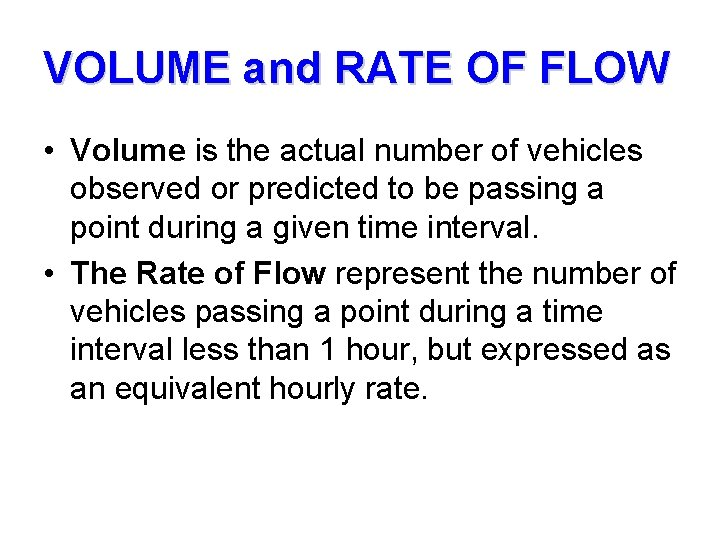VOLUME and RATE OF FLOW • Volume is the actual number of vehicles observed or predicted to be passing a point during a given time interval. • The Rate of Flow represent the number of vehicles passing a point during a time interval less than 1 hour, but expressed as an equivalent hourly rate.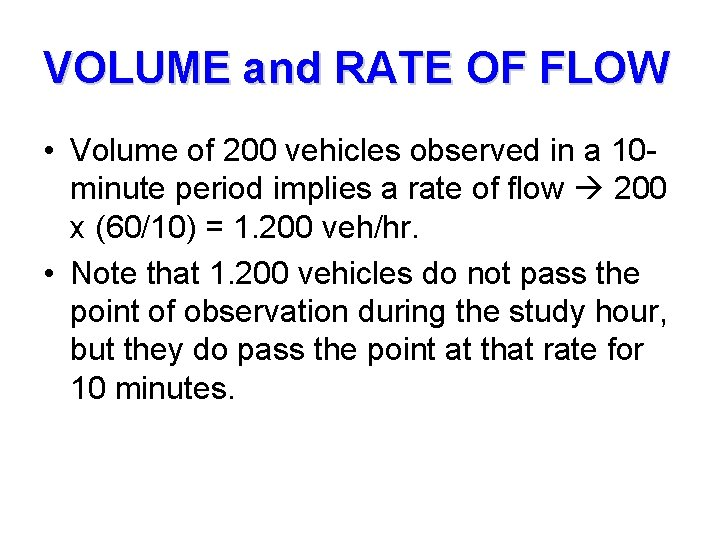VOLUME and RATE OF FLOW • Volume of 200 vehicles observed in a 10 minute period implies a rate of flow 200 x (60/10) = 1. 200 veh/hr. • Note that 1. 200 vehicles do not pass the point of observation during the study hour, but they do pass the point at that rate for 10 minutes.VOLUME and RATE OF FLOW Time Period Volume (vehicles) 4: 00 – 4: 15 700 4: 16 – 4: 30 812 4: 30 – 5: 00 1. 635 Total 3. 147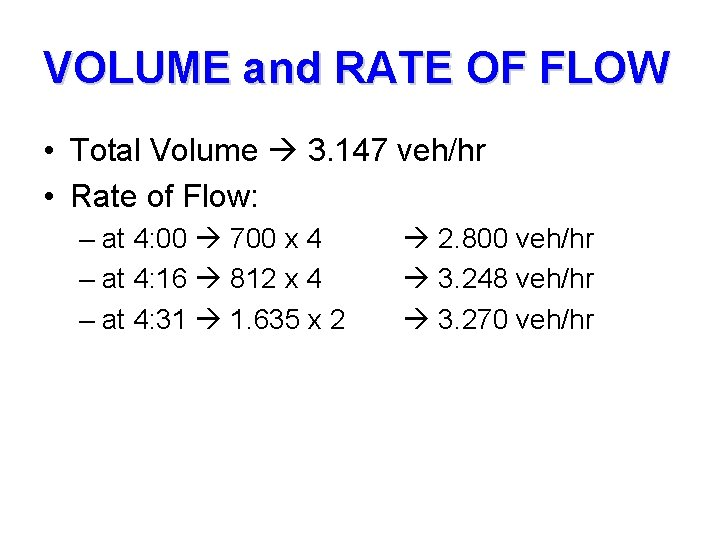VOLUME and RATE OF FLOW • Total Volume 3. 147 veh/hr • Rate of Flow: – at 4: 00 700 x 4 – at 4: 16 812 x 4 – at 4: 31 1. 635 x 2 2. 800 veh/hr 3. 248 veh/hr 3. 270 veh/hrDENSITY • Number of vehicles occupying a given length of lane or roadway, averaged over time, usually expressed as vehicles per km (veh/km) • q=vxk • q = rate of flow (veh/hr) • v = average travel speed (kph) • k = average density (veh/km)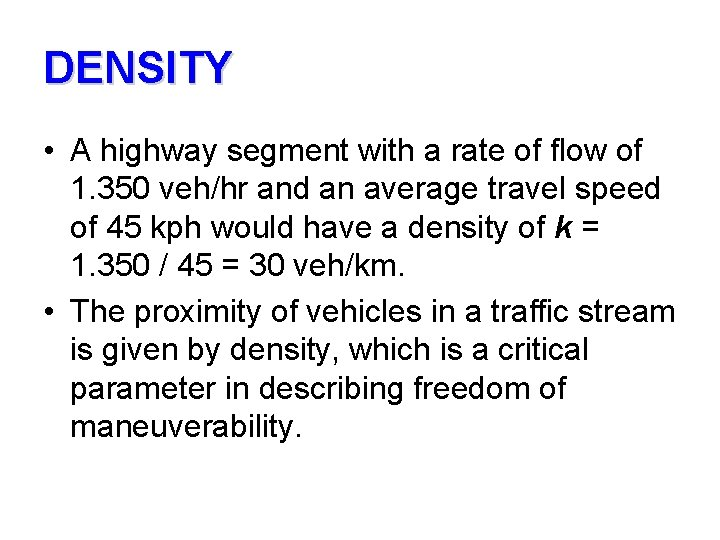DENSITY • A highway segment with a rate of flow of 1. 350 veh/hr and an average travel speed of 45 kph would have a density of k = 1. 350 / 45 = 30 veh/km. • The proximity of vehicles in a traffic stream is given by density, which is a critical parameter in describing freedom of maneuverability.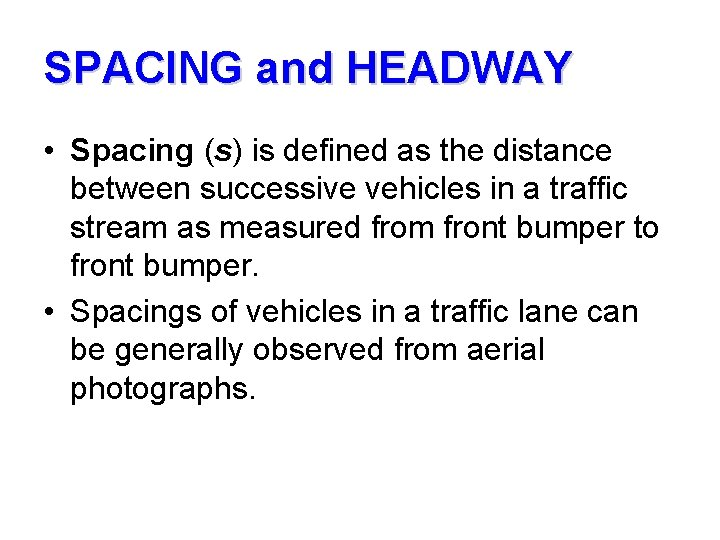SPACING and HEADWAY • Spacing (s) is defined as the distance between successive vehicles in a traffic stream as measured from front bumper to front bumper. • Spacings of vehicles in a traffic lane can be generally observed from aerial photographs.SPACING and HEADWAY • Headway is the corresponding time between successive vehicles as they pass a point on a roadway. • Headways of vehicles can be measured using stopwatch observations as vehicles pass a point on a lane.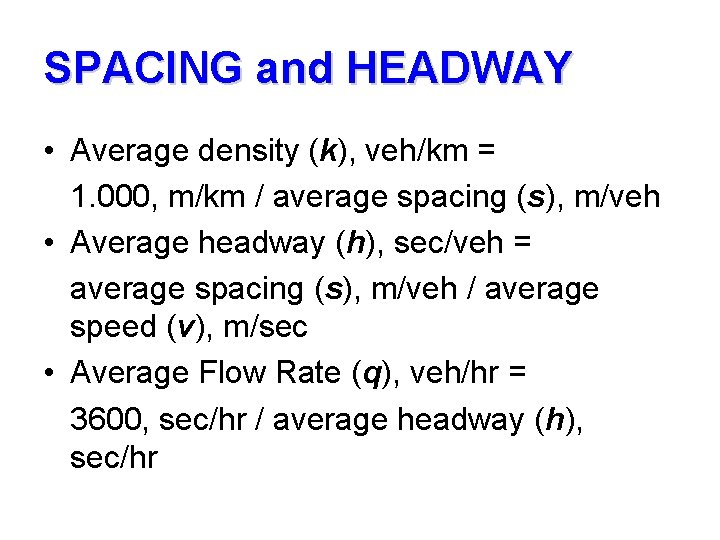SPACING and HEADWAY • Average density (k), veh/km = 1. 000, m/km / average spacing (s), m/veh • Average headway (h), sec/veh = average spacing (s), m/veh / average speed (v), m/sec • Average Flow Rate (q), veh/hr = 3600, sec/hr / average headway (h), sec/hrLANE OCCUPANCY • Lane occupancy is a measure used in freeway surveillance. • If one could measure the lengths of vehicles on a given roadway section and compute the ratio • R could be divided by the average length of vehicle to give an estimate of the density (k)CLEARANCE AND GAP • Correspond to parameters of spacing (m) and headway (sec) Clearance (m) L (m) Gap (sec) Spacing (m) or Headway (sec)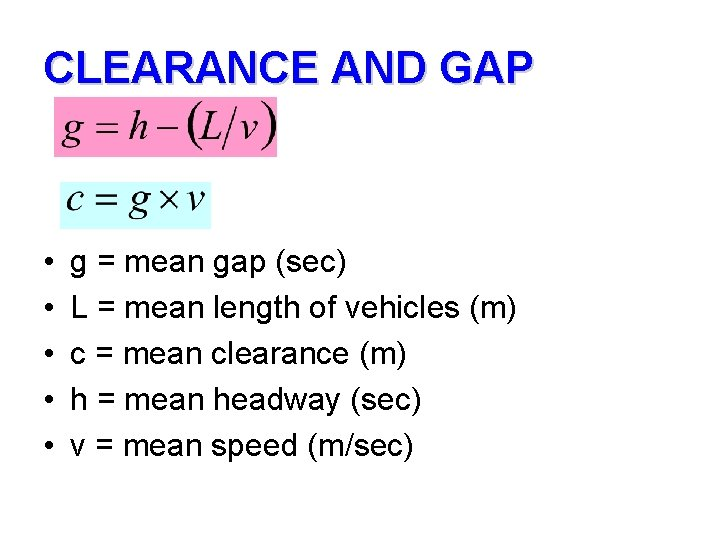CLEARANCE AND GAP • • • g = mean gap (sec) L = mean length of vehicles (m) c = mean clearance (m) h = mean headway (sec) v = mean speed (m/sec)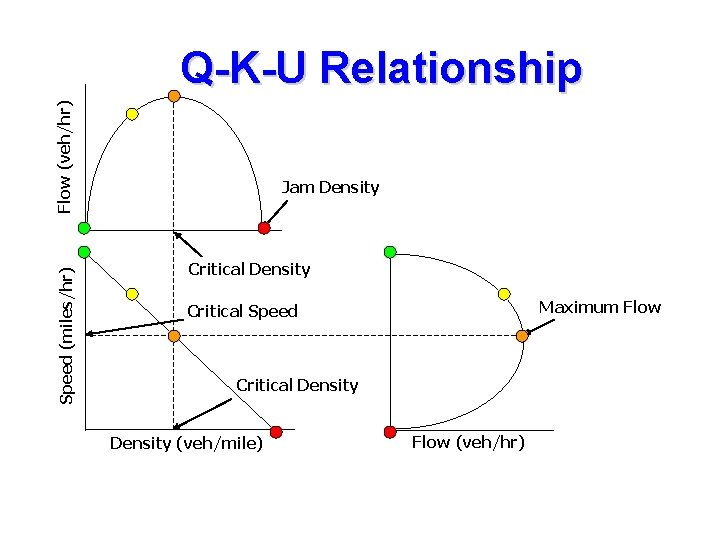Speed (miles/hr) Flow (veh/hr) Q-K-U Relationship Jam Density Critical Density Maximum Flow Critical Speed Critical Density (veh/mile) Flow (veh/hr)SPEED, FLOW, AND DENSITY RELATIONSHIP • q=uxk q = Ak – Bk 2 q = (A – Bk) x k • u = A – Bk • k=(A–u)/B • q = u x [( A – u ) / B] q=[ux(A–u)]/B • M=1/k • q = (A/M) – (B/M 2)Traffic DataSPEED, FLOW, AND DENSITY RELATIONSHIP Correlations: Q, U, K U K Q 0. 133 0. 180 U 0. 119 0. 228 -0. 921 0. 000 Best Negative Correlation!!! Cell Contents: Pearson correlation P-Value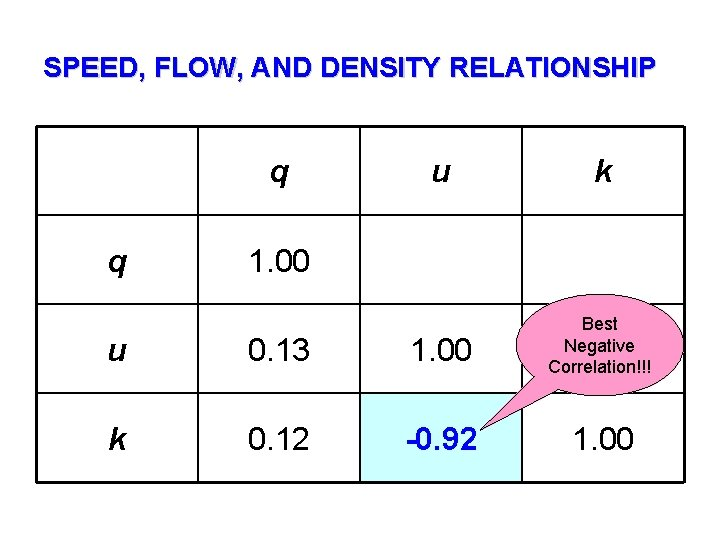SPEED, FLOW, AND DENSITY RELATIONSHIP q q u k 1. 00 u 0. 13 1. 00 Best Negative Correlation!!! k 0. 12 -0. 92 1. 00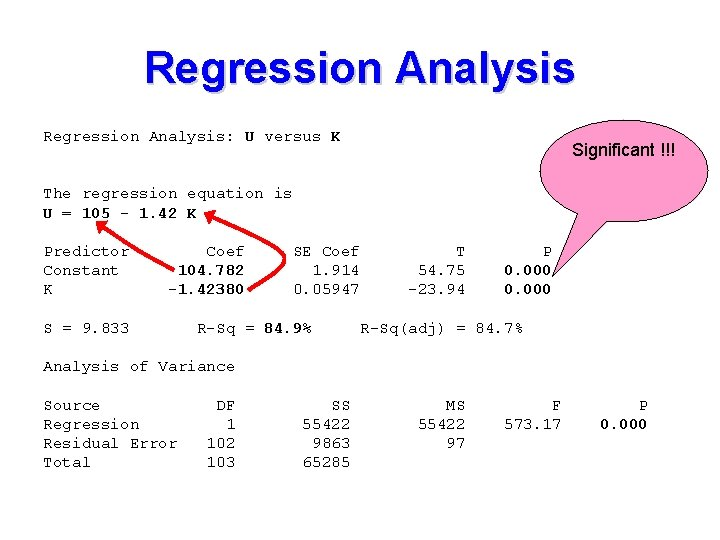Regression Analysis: U versus K Significant !!! The regression equation is U = 105 - 1. 42 K Predictor Constant K Coef 104. 782 -1. 42380 S = 9. 833 SE Coef 1. 914 0. 05947 R-Sq = 84. 9% T 54. 75 -23. 94 P 0. 000 R-Sq(adj) = 84. 7% Analysis of Variance Source Regression Residual Error Total DF 1 102 103 SS 55422 9863 65285 MS 55422 97 F 573. 17 P 0. 000SPEED, FLOW, AND DENSITY RELATIONSHIP • A = 104, 78 • B = -1, 4238 • u = A + Bk 104, 78 - 1, 4238 k • q = Ak – Bk 2 104, 78 k + 1, 4238 k 2 • q = [u x (A-u)]/ B [u x (104, 78 – u)] / 1, 4238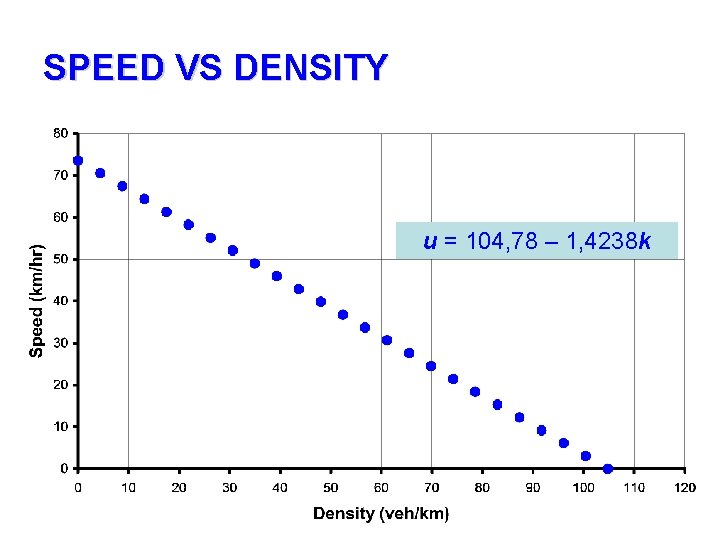SPEED VS DENSITY u = 104, 78 – 1, 4238 k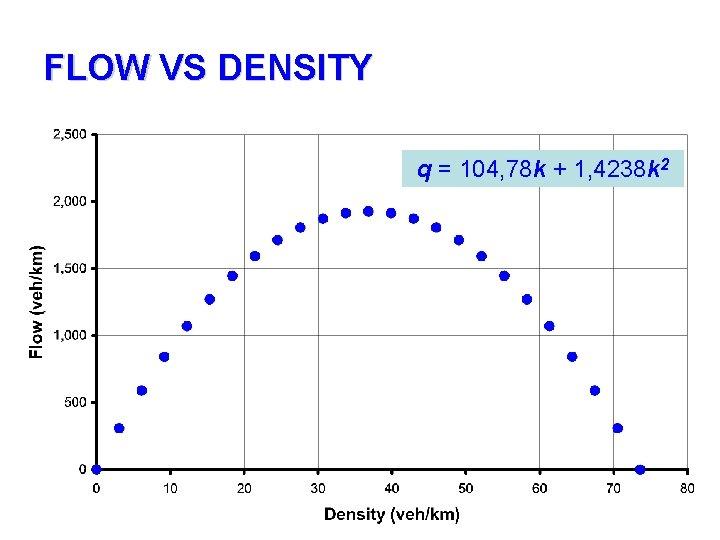FLOW VS DENSITY q = 104, 78 k + 1, 4238 k 2SPEED VS FLOW q = [u x (104, 78 -u)] / 1, 4238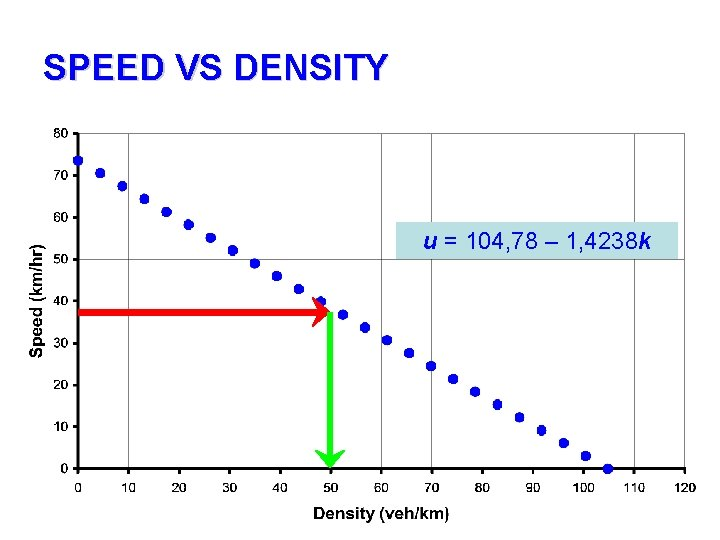SPEED VS DENSITY u = 104, 78 – 1, 4238 kFLOW VS DENSITY q = 104, 78 k + 1, 4238 k 2SPEED VS FLOW q = [u x (104, 78 -u)] / 1, 4238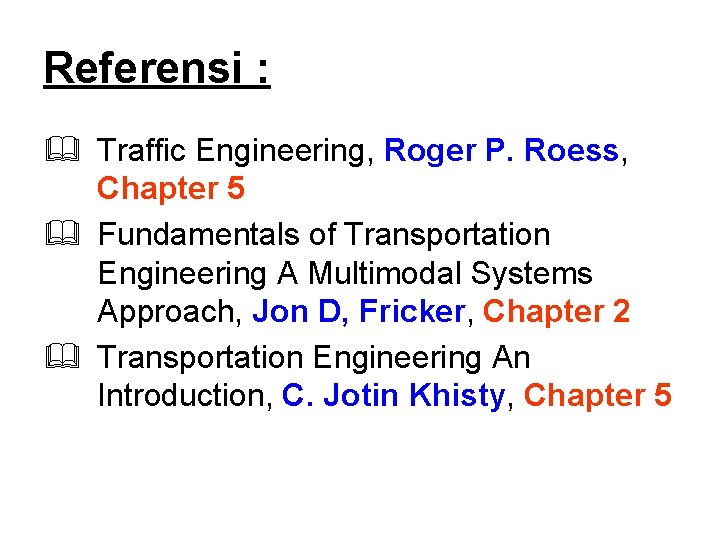Referensi : & Traffic Engineering, Roger P. Roess, Chapter 5 & Fundamentals of Transportation Engineering A Multimodal Systems Approach, Jon D, Fricker, Chapter 2 & Transportation Engineering An Introduction, C. Jotin Khisty, Chapter 5